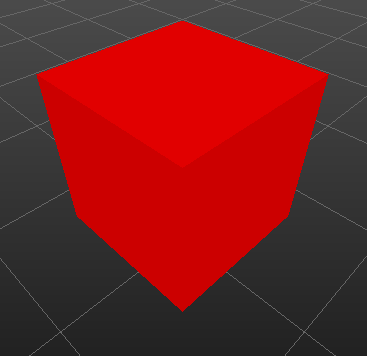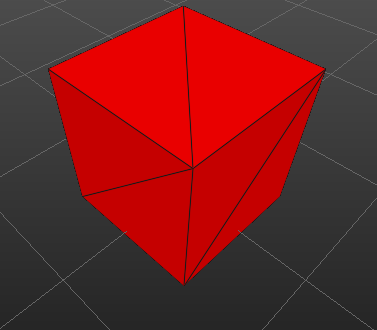## Saturday, September 10, 2011

### Single-Pass Wireframe Rendering

It came time for me to add a wireframe to my mesh and just when I was about to do the standard two-pass approach of rendering out my mesh faces and then rendering my wireframe with GL_LINES over that, I came across Single-Pass Wireframe Rendering, a simple idea for rendering my faces and lines in just one pass.  The idea, to put it simply, is to add some smarts to the fragment code so when it's rendering fragments close to the sides of a face, it blends in an edge color.  The paper gives several reasons why this is a better approach including better performance and some really cool added abilities.  The best part is it's very easy to add to existing code without much modification.

My code already had a basic vertex/fragment shader for doing some basic lighting and I just needed to add geometry shader in between that could add an attribute to each vertex specifying how far the fragment would be from the edge in screen space.

Here's the geometry shader taken almost straight from their full paper off their site...

``` #version 120 #extension GL_EXT_gpu_shader4 : enable #extension GL_EXT_geometry_shader4 : enable varying in vec3 vertWorldPos; varying in vec3 vertWorldNormal; varying out vec3 worldNormal; varying out vec3 worldPos; uniform vec2 WIN_SCALE; noperspective varying vec3 dist; void main(void) { // taken from 'Single-Pass Wireframe Rendering' vec2 p0 = WIN_SCALE * gl_PositionIn.xy/gl_PositionIn.w; vec2 p1 = WIN_SCALE * gl_PositionIn.xy/gl_PositionIn.w; vec2 p2 = WIN_SCALE * gl_PositionIn.xy/gl_PositionIn.w; vec2 v0 = p2-p1; vec2 v1 = p2-p0; vec2 v2 = p1-p0; float area = abs(v1.x*v2.y - v1.y * v2.x); dist = vec3(area/length(v0),0,0); worldPos = vertWorldPos; worldNormal = vertWorldNormal; gl_Position = gl_PositionIn; EmitVertex(); dist = vec3(0,area/length(v1),0); worldPos = vertWorldPos; worldNormal = vertWorldNormal; gl_Position = gl_PositionIn; EmitVertex(); dist = vec3(0,0,area/length(v2)); worldPos = vertWorldPos; worldNormal = vertWorldNormal; gl_Position = gl_PositionIn; EmitVertex(); EndPrimitive(); } ```

If you're already familiar with the vertex/fragment shader pipeline, which has been around quite a few years longer than the geometry shader, you'll recognize nothing is too out of the ordinary. It takes a world position and normal, which is basically just passed off to the fragment shader for lighting purposes. Although I've done quite a bit of GLSL, this was my first attempt at using a geometry shader, and once I learned the basic idea, I found it pretty intuitive.

First, there are varying inputs from the vertex shader that come in as arrays--one element for each vertex. The names have to match up, so for the in vec3 vertWorldPos attribute, there must be a corresponding out vec3 vertWorldPos designated in the vertex shader. The exception to this is predefined variables like gl_Position, which comes in as gl_PositionIn[]. Not sure why the OpenGL designers decided to add those two letters, but whatever.

WIN_SCALE is the screen size, which we multiply be the vertex position XY. This takes our vertex positions in viewport space and converts them to screen space since we want to measure our distances in pixels in the fragment shader. That's followed by some basic trig to calculate the area of the triangle, which is used to find the altitude of each vertex (the closest distance to the opposing edge). Because the altitude is already in screen space, the noperspective keyword is added to disable perspective correction.

The geometry shader is responsible for actually creating the primitives via the EmitVertex() and EndPrimitive() functions. When EmitVertex() function is called, it sends a vertex down the pipeline with attributes based on whatever the out attributes happen to be set to at the time. EndPrimitive() just tells OpenGL that the vertices already sent down are ready to be rasterized as a primitive.

The geometry shader can actually create additional geometry on the fly, but it comes with some caveats. We must designate in our C++ code an upper bound of how many vertices we might want to create. This geometry shader doesn't create any additional geometry, but it's still useful as it provides knowledge of the neighboring vertices to calculate the outgoing vertex altitudes.

Setting Up the Geometry Shader
Using Qt, the geometry shader is compiled just like the vertex and fragment shader.
``` QGLShader* vertShader = new QGLShader(QGLShader::Vertex); vertShader->compileSourceCode(vertSource); QGLShader* geomShader = new QGLShader(QGLShader::Geometry); geomShader->compileSourceCode(geomSource); QGLShader* fragShader = new QGLShader(QGLShader::Fragment); fragShader->compileSourceCode(fragSource); QGLShaderProgramP program = QGLShaderProgramP(new QGLShaderProgram(parent)); program->addShader(vertShader); program->addShader(geomShader); program->addShader(fragShader); ```

The only other adjustment is when we bind our shader. Because the geometry shader can create more geometry than inputted, it requires giving OpenGL a heads up of how much geometry you might create. You don't necessarily have to create all the vertices you allocate, but it's just a heads up for OpenGL. You can all also configure the geometry shader to output a different type of primitive than inputted like creating GL_POINTS from GL_TRIANGLES. Because this geometry shader is just taking a triangle in and outputting a triangle, we can just set the number of outgoing vertices to the number going in. GL_GEOMETRY_INPUT_TYPE, GL_GEOMETRY_OUTPUT_TYPE, and GL_GEOMETRY_VERTICES_OUT need to be specified prior to linking the shader.

``` QGLShaderProgram* meshShader = panel->getShader(); // geometry-shader attributes must be applied prior to linking meshShader->setGeometryInputType(GL_TRIANGLES); meshShader->setGeometryOutputType(GL_TRIANGLES); meshShader->setGeometryOutputVertexCount(numTriangles*3); meshShader->bind(); meshShader->setUniformValue("WIN_SCALE", QVector2D(panel->width(),panel->height())); meshShader->setUniformValue("objToWorld", objToWorld); meshShader->setUniformValue("normalToWorld", normalToWorld); meshShader->setUniformValue("cameraPV", cameraProjViewM); meshShader->setUniformValue("cameraPos", camera->eye()); meshShader->setUniformValue("lightDir", -camera->lookDir().normalized()); ```

We also need to modify the fragment shader to take this distance variable into account to see if our fragment is close to the edge.

``` #version 120 #extension GL_EXT_gpu_shader4 : enable varying vec3 worldPos; varying vec3 worldNormal; noperspective varying vec3 dist; uniform vec3 cameraPos; uniform vec3 lightDir; uniform vec4 singleColor; uniform float isSingleColor; void main() { // determine frag distance to closest edge float nearD = min(min(dist,dist),dist); float edgeIntensity = exp2(-1.0*nearD*nearD); vec3 L = lightDir; vec3 V = normalize(cameraPos - worldPos); vec3 N = normalize(worldNormal); vec3 H = normalize(L+V); vec4 color = isSingleColor*singleColor + (1.0-isSingleColor)*gl_Color; float amb = 0.6; vec4 ambient = color * amb; vec4 diffuse = color * (1.0 - amb) * max(dot(L, N), 0.0); vec4 specular = vec4(0.0); edgeIntensity = 0.0; // blend between edge color and normal lighting color gl_FragColor = (edgeIntensity * vec4(0.1,0.1,0.1,1.0)) + ((1.0-edgeIntensity) * vec4(ambient + diffuse + specular)); } ```

And that's it! It takes a bit more work to get it working with quads, but once done you can do some pretty wild and awesome tricks as shown on the author's wireframe site.BeforeAfter

And here's the vertex shader for reference, but as you can see it's quite simple because a bit of the processing it's did has been moved to the geometry shader. Nothing here really even has to do with the edge rendering.

``` #version 120 #extension GL_EXT_gpu_shader4 : enable in vec3 vertex; in vec4 color; in vec3 normal; varying vec3 vertWorldPos; varying vec3 vertWorldNormal; uniform mat4 objToWorld; uniform mat4 cameraPV; uniform mat4 normalToWorld; void main() { vertWorldPos = (objToWorld * vec4(vertex,1.0)).xyz; vertWorldNormal = (normalToWorld * vec4(normal,1.0)).xyz; gl_Position = cameraPV * objToWorld * vec4(vertex,1.0); gl_FrontColor = color; } ```

jodag said...
This comment has been removed by the author.
Unknown said...

Thank you!
I used this in my university assignment, and it works great!
https://www.dropbox.com/s/10j2q2l40xz4ctm/CessnaWireframe.png?dl=0

Unknown said...

I know this is an old post, but I've been bashing my head against this for a few days now, and thought someone might also need this. :)
If you the geometry shader above, you will end up with incorrect distance values in some cases, as you can see here: https://gamedev.stackexchange.com/questions/136915/geometry-shader-wireframe-not-rendering-correctly-glsl-opengl-c
This is because the transformation from world to screen space (vec2 p0 = WIN_SCALE * gl_PositionIn.xy/gl_PositionIn.w;) is wrong. You need to multiply by HALF of the size of your viewport, because (0;0) is in the middle. Technically to get screen coordinates you'd also have to add vec2(1,1) to them first and also translate them based on the viewport location, but in this case only the scale matters.